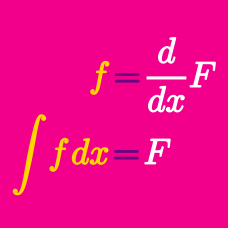Calculus

# Integrals - Problem Solving

Evaluate $\int _{ 0 }^{ \frac{\pi}{4} }{ \frac{21 \cos^2 x}{\sin x + \cos x} } dx + \int _{ \frac{\pi}{4} }^{ 0 }{ \frac{21 \sin^2 x}{\sin x + \cos x} } dx.$

If $$f(x)=\int \frac{\left(\sqrt{x}-1\right)^2}{\sqrt{x}} dx$$ and $$\displaystyle f(1)=\frac{5}{3},$$ what is the value of $$3f(16)?$$

Let $$\displaystyle f(x)=\int_{0}^{x}{\sqrt{7+6{t}^{2}}dt.}$$ Then what are the real roots of the equation ${x}^{2}-\frac{df(x)}{dx}=0?$

If $$f(m)$$ is the number of the points crossed by the curve $$y=x^2-mx+m$$ and the $$x$$-axis, what is $$\displaystyle \int_{7}^{12} f(m) \,dm ?$$

Suppose $$f(x)$$ and $$F(x)$$ are polynomial functions such that $F'(x) = f(x) \mbox{ and } F(x)=xf(x)-4x^3+x^2.$ If $$f(0)=6,$$ what is $$f(x)?$$

×